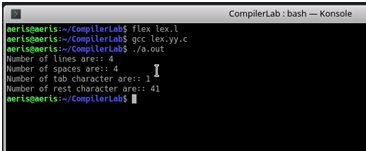# LEX Code to count the number of lines, space, tab-meta character and rest of characters in a given Input pattern

In this article, we going to learn how to create LEX program to analysis how many line, space, tab character and other character are present in given input file?
Submitted by Ashish Varshney, on March 28, 2018

For achieve to this task we create some rules in rule section in the code.

Example:

```    Input: a b c d

Output:
Number of lines: 1
Number of spaces: 3
Number of character: 4
```

Code:

```/*Definition Section*/
%{
#include<stdio.h>
#include<stdlib.h>
int count=0,space=0,tcount=0,rcount=0;  /*Global variables*/
%}

/*Rule Section*/
%%
/*Increase the count whenever encounter newline.*/
\n count++;
/* Increase the value of space variable whenever encounter " ".*/
" " space++;
/* Increase the value of tcount variable whenever encounter  tab .*/
\t tcount++;
/* Otherwise, Increase the value of rcount variable.*/
[^\t" "\n] rcount++;
. ;
%%

/*Auxiliary function*/
/*Driver function*/
int main(void)
{
yyin=fopen("q1.txt","r");
/*call the yylex function.*/
yylex();
/*print the number of lines.*/
printf("Number of lines are:: %d\n",count);
/*print the number of spaces.*/
printf("Number of spaces are:: %d\n",space);
/*print the number of tab spaces.*/
printf("Number of tab character are:: %d\n",tcount);
/*print the number of other character.*/
printf("Number of rest character are:: %d\n",rcount);
return 0;
}

/*call the yywrap function*/
int yywrap()
{return 1;}
```

Input

```    q1.txt(file)
include  helpedu   website
btech
section CSA
a	b
```

Output